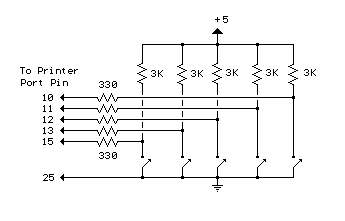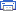# Reading Data From The Parallel Port Electronic Schematics > PC related > Reading Data From The Parallel Port

The diagram below shows 5 switches connected to the 5 input lines of the parallel port. An external 5 volt power supply is used to provide high logic levels to the input pins when the switches are open. Three 1.5 volt batteries in series can be used to obtain 4.5 volts which is close enough. The 330 ohm resistors in series with the port connections provide some protection in case a connection is made to the wrong pin. If you are sure of the connections, the 330 ohm resistors can be left out and the switches connected directly to the input pins. The negative side of the power supply should be connected to the ground point, or any pin from 18 to 25.The following short QBasic program can be used to read the state of the switches. QBASIC.EXE can be found in the "OLDMSDOS" directory of the Windows 95/98 CD Rom. Note that there are three possible printer port address that correspond to LPT1, LPT2 and LPT3 and LPT1 is usually the one to use which is at address decimal 889. The program waits for the user to press the enter key before reading the state of the 5 input lines. The state of the 5 lines is received as a single 8 bit number between 0-255 which is stored as the value of (V). Each switch input represents a decimal value of 8,16,32,64 and 128 which correspond to pins 15,13,12,10 and 11. The last 3 bits (1,2 and 4) are not used and should return a high level, so the value received with all switches open should be 1+2+4+8+16+32+64=127. If a switch is closed and the input is at ground, the value will be 0 except for pin 11 which is inverted and yields a value of 128 and 0 when high, so the value received when all switches are closed should be 1+2+4+128=135.
-----------------------------------------------------------------------

CLS
DEFINT A-Z
PRINT "Press the enter key to read printer port pins (15,13,12,10,11)"
PRINT "A (0) reading indicates the pin is at ground level, (1) indicates"
PRINT "the pin is at a high level or unterminated."
INPUT A\$
PRINT V
P11 = 1
IF V > 127 THEN P11 = 0: V = V - 128
IF V > 63 THEN P10 = 1: V = V - 64
IF V > 31 THEN P12 = 1: V = V - 32
IF V > 15 THEN P13 = 1: V = V - 16
IF V > 7 THEN P15 = 1
PRINT
PRINT "Pin 15 ="; P15
PRINT "Pin 13 ="; P13
PRINT "Pin 12 ="; P12
PRINT "Pin 10 ="; P10
PRINT "Pin 11 ="; P11
END

Title: Reading Data From The Parallel Port
electronic circuit
Source: unknown
Published on: 2007-07-21
Print version: